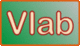online_pump_pipe_system:  Operating discharge and head in a pump-pipe systemDefinition sketch of a pump-pipe system

 Conservation of energy: E1 + hp = E2 + [V2/(2g)] [Ke + ∑Kb + KE + f(L/D)] hp = ΔE + [Q2/(2gA2)] [Ke + ∑Kb + KE + f(L/D)] hp = ΔE + [Q2/(2g(πD2/4)2] [Ke + ∑Kb + KE + f(L/D)] hp = ΔE + [16/(2gπ2D4)] [Ke + ∑Kb + KE + f(L/D)] Q2 a = ΔE b = [16/(2gπ2D4)] [Ke + ∑Kb + KE + f(L/D)] System head-discharge relation: hp = a + b Q2 Type 1 head-discharge relation Pump head-discharge relation: H = c - d Q2 System operating discharge: Qoper = [(c - a)/(b + d)]1/2 System operating head: Hoper = c - d [(c - a)/(b + d)]

Thank you for running online_pump_pipe_system.